Designing a 3+3/PC trial

Precautionary Coherence and the ‘3+3/PC’ Design

Perhaps you had been planning to conduct a phase 1 dose-finding trial using a dose-escalation design built upon any of the usual, one-size-fits-all methods such as the 3+3, CRM, EWOC, mTPI, BOIN, etc. But then somehow you stumbled upon (Norris 2017c), and learned:

• that dose-escalation designs are fundamentally unethical,
• that a few modifications can convert the inveterate ‘3+3’ dose-escalation design into an efficient ‘3+3/PC’ dose-titration design
• and that 3+3/PC outperforms even the fancy ‘model-based’ methods, proving definitively that

3+3 is not the problem; 1-size-fits-all dose finding is the problem!

In that case … this vignette is for you. Here, we will work stepwise through the process of developing a 3+3/PC design, and simulating its performance.

Optimal-Dose Heterogeneity

Your first step in designing a dose-finding trial should be to articulate your expectations regarding the heterogeneity of optimal dosing in your patient population. In what follows, I’ll assume we’re working under a maximum tolerated dose (MTD) heuristic, so that we denote the optimal dose for individual i as MTDi (Norris 2017a). Formally, the most straightforward way to describe MTDi heterogeneity is with a distribution:

# You might find it easiest to think in terms of CV and median:
CV <- 0.7    # coefficient of variation of MTDi
MTDi50 <- 75 # median MTDi (i.e., 50th percentile)
# But R's Gamma distribution will expect that information
# expressed in terms of 'shape' and 'scale' parameters:
shape <- CV^-2
scale <- MTDi50/qgamma(0.5, shape=CV^-2)
# Plot:
MTDi <- 0:200
dens <- dgamma(MTDi, shape=shape, scale=scale)
xyplot(dens ~ MTDi, type = "l"
, xlab = expression(MTD[i]*' [mg/kg]')
, ylab = "density")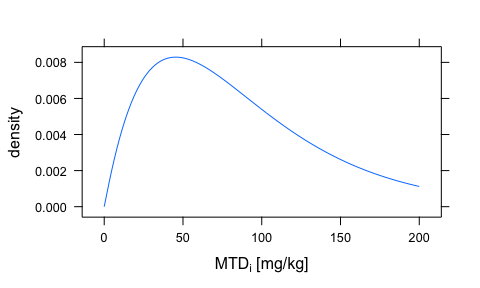A postulated distribution of MTDi.

A density as plotted above has a natural intuitive appeal, in part because it shows clearly the central tendency of the distribution, and depicts rare individuals extending into its ‘tail’ to the right. (To appreciate what motivates the particular choice of the Gamma distribution here, please see (Norris 2017b).)

More directly useful than this density, however, is the corresponding cumulative distribution:

F <- pgamma(MTDi, shape=shape, scale=scale)
xyplot(F ~ MTDi, type = "l"
, xlab = expression(MTD[i]*' [mg/kg]')
, ylab = "Cumulative Distribution"
, panel = function(...){
panel.xyplot(...)
panel.refline(h=0.5)
panel.refline(v=MTDi50)
})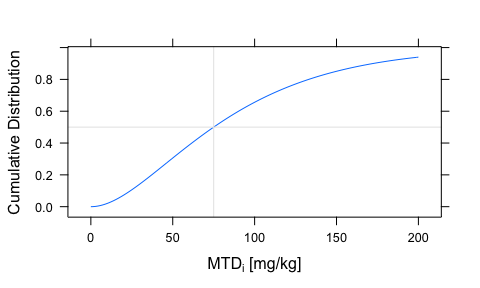A postulated cumulative distribution of MTDi.

Whatever a cumulative distribution sacrifices in terms of direct visual appeal, it makes up for with its practical utility. As shown by the crosshairs superimposed on this plot, you can read straight off this plot the median MTDi of 75 mg/kg that you had posited above.

Before moving on, let’s introduce one final modification to this cumulative distribution, which should look familiar to the oncology trialist:

xyplot((1-F) ~ MTDi, type = "l"
, xlab = "Dose [mg/kg]"
, ylab = "Fraction Tolerant")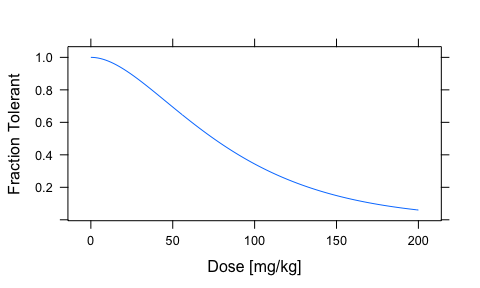The ‘dose-survival curve’ implied by the posited distribution of MTDi.

As you can see, we’ve simply flipped the cumulative distribution vertically. (To appreciate this in the code, note that the plotting formula has changed from F ~ MTDi to (1-F) ~ MTDi.)

By inverting the customary mathematical treatment, we avoid an unnatural inversion of our clinical language. Thus, we can now speak about what fraction of patients will tolerate any given dose. For obvious reasons, (Norris 2017c) calls this a ‘dose-survival curve’.

Precautionary Coherence and Dose Ranging

Precautionary Coherence

The precautionary coherence (PC) principle introduced in (Norris 2017c) requires that “a PC trial conducts escalation only through titration.” Please see that paper or this lay explainer for a fuller discussion. To appreciate the ethical content of the PC principle in an especially vivid way, you might try imagining yourself in the trialist’s place in this scenario.

Dose Ranging in General

As with so much of the terminology in the thoroughly muddled field of dose-finding methodology, the term ‘dose ranging’ is subject to a variety of confusing usages. What I find most useful about this term is that the word range underscore the uncertainty inherent in optimal dosing, helping to debunk the false sense of certainty implied by usage such as ‘the’ MTD (Norris 2017a).

In my view, a dose-ranging study in general opens with a proposed range of doses that researchers initially deem reasonable to try in participants. As the study proceeds, researchers learn from the accumulating data, and revise their proposed range. For some historical background on dose ranging—albeit in a chronic-disease context—see (Sheiner, Beal, and Sambol 1989).

3+3/PC as a Dose Ranging Design

As you will see, the 3+3/PC is very much a dose-ranging design in this sense. It begins by laying out a discrete set of (a half-dozen or so) doses that cover some range the trialists consider reasonable. (The trialists may base their considerations on preclinical studies, for example.) At the low end of this range, the trialists will posit a dose they feel reasonably sure is safe. Conversely, at the top end of the range, the trialists will include a dose they think should suffice to deliver the postulated benefits of the drug. As the study proceeds, the trialists may discover that the dose range they initially ‘staked out’ requires modification. They might realize they can safely pull up some of the stakes at the lower end, if most patients easily tolerate these doses. The same applies at the high end, for the opposite reason: some of the higher doses may prove toxic to a great majority of patients.

Dose Ranging in Our Example

Returning to our example study, suppose that you decide to explore 7 doses spanning the range from 25 to 200 mg/kg. The ends of this range are a factor of 8 apart, rendering a geometric sequence highly suitable:

doses <- 25 * (200/25)^(0:6/6) # geometric sequence 25..200
doses <- round(doses/5)*5      # round to the nearest 5 mg/kg
names(doses) <- 1:7            # label the doses 1..7
doses
##   1   2   3   4   5   6   7
##  25  35  50  70 100 140 200

For context, let’s see these 7 doses overplotted against our posited MTDi distribution:

xyplot(dens ~ MTDi, type = "l"
, xlab = expression(MTD[i]*' [mg/kg]')
, ylab = "density"
, scales = list(x=list(log=FALSE, at=doses)))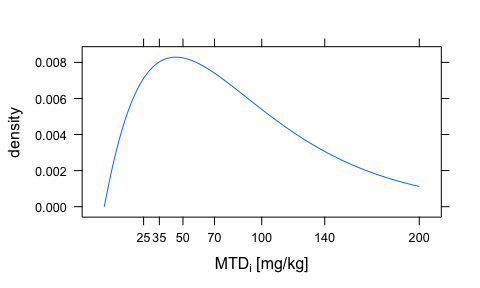Discrete doses spanning our anticipated MTDi distribution.

Re-plotting over a logarithmically scaled dose axis, we see our geometric sequence of doses becomes ‘evenly spaced’. Furthermore, the evident ‘rectification’ of the Gamma distribution’s skewness indicates that our choice of Gamma distribution may have been appropriate to the logarithmic span of our uncertainty about dosing:

xyplot((dens*MTDi) ~ MTDi, type = "l"
, xlab = expression(MTD[i]*' [mg/kg]')
, xlim = c(10, 200)
, ylab = expression("density of",log(MTD[i]))
, scales = list(x=list(log=TRUE, at=doses)))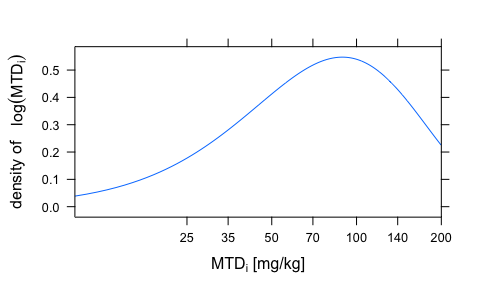Discrete doses spanning our anticipated MTDi distribution, with logarithmic dose scaling.

Likewise, this same scaling somewhat ‘straightens out’ our dose-survival curve:

xyplot((1-F) ~ MTDi, type = "l"
, xlab = "Dose [mg/kg]"
, xlim = c(20, 200)
, ylab = "Fraction Tolerant"
, scales = list(x=list(log=TRUE, at=doses)))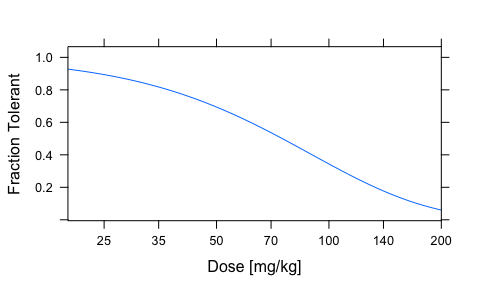Discrete doses spanning our anticipated dose-survival curve, with logarithmic dose scaling.

Of note, the increases between doses are by a factor of $$\sqrt{200/25} = \sqrt{2} \approx 1.41$$, matching the 40% “dose-step increments” for which (Simon et al. 1997) provides some precedent.

Having decided what doses to trial, let’s see what we might learn if we enrolled 24 participants in a 3+3/PC titration study that runs for 10 dosing intervals.

N <- 24
set.seed(2019) # setting RNG seed makes sim reproducible
trial <- new("DE", doses=doses,
MTDi=rgamma(N, shape=shape, scale=scale),
units="mg/kg")
trial <- titration(trial, periods=10)
viz <- plot(trial)

Introduction to the Dose-Titration Diagram

Like the dose-titration design it depicts, this diagram is fairly rich. Exploring the interactivity of this diagram seems to me essential for developing a full understanding of it. (By contrast, diagrams for dose-escalation designs are typically quite austere. You can find examples of those in (Cheung 2005) or in this online presentation by the same author.)

Following a custom first established in explosives research (Dixon and Mood 1948), X’s represent toxicities and the O’s tolerated doses. To compensate for the ‘crowding’ caused by titration, I have introduced several visual aids to help follow individuals as they percolate through this diagram. A cycle of 4 (colorblind-friendly) colors helps discriminate between the cohorts enrolling in successive periods. Left-to-right positioning distinguishes the 3 individual participants within each cohort. Furthermore, when you hover over an individual’s marker, a gray ‘trace’ tracks that participant’s titration course. If you hover over participant 11, for example, you will see that she is the middle participant in the magenta-colored cohort that enrolled in period 4. (Notice that the cycle of colors repeats, so that the final cohort enrolling in period 8—i.e., participants 22, 23 and 24—also get magenta markers.)

3+3/PC Rules

The simulated trial realization depicted here illustrates nearly all of the rules described on page 3 of (Norris 2017c):

• PC rule

In this diagram, escalation (i.e., trying a new higher dose) occurs only through titration. Thus, the period-2 escalation to dose level 2 is accomplished by titrating the (green) period-1 cohort upward. In standard dose-escalation trials, which violate the PC principle, the (orange) second cohort (participants 4–6) would have leapfrogged the first and enrolled straight into dose level 2. Worse, the (purple) third cohort would have enrolled straight into dose level 3. Why is that so bad? Notice that participant 9 has an MTDi of 23.8 mg/kg, and so cannot even tolerate the 25 mg/kg of dose level 1. How much worse would it have been for him to enroll straight into dose level 3! (This explains the ‘precautionary’ in PC.)

• cohort rule

To preserve the ‘3+3’ spirit of the 3+3/PC design, we titrate upward to a new dose only when a cohort of 3 or more participants has ‘accumulated’ at the next-lower dose. This is why no escalation occurs in period 4. Because participant 2 had a DLT in period 3, only participants 1 and 3 were eligible to escalate to the not-yet-tried dose level 4. Accordingly, they had to wait until (orange) participants 4–6 joined them in period 4, providing a ‘quorum’ for escalation in period 5.

• bypass rule

The 3+3/PC design accumulates DLTs into the dose-survival curve on the right-hand side of the diagram. (In what follows, I will discuss that curve as if you were turning your head 90 degrees to the left to view it.) Click the period divider between periods 5 and 6, to see what the dose-survival curve looked like at the end of period 5. As you’ll see, the (dashed) 80% confidence band around the (solid) Kaplan-Meier-style dose-survival plot sits just above the 0.8 threshold at this point. (The lower bound in fact hovers at 0.8013.) According to the bypass rule, we take this to indicate that this dose can be abandoned as a starting dose for newly-enrolled participants. This is why the (orange) 6th cohort (participants 16–18) bypass dose level 1 to enroll straight into dose level 2. Note that a similar thing happens again in period 7, resulting in the further bypassing of dose 2.

• reduction rule & exit convention

After experiencing a DLT, a participant drops to the next-lower dose. Thus participant 2, having experienced a DLT at dose level 3, will drop back to dose level 2 in subsequent periods. Since this is ‘understood’, we need not carry particpant 2 forward in this already quite busy diagram. Thus, the ‘exit convention’ applies, and we can drop this participant’s marker in period 4 and subsequently. (Of course, this does not mean we forget the DLT. The DLT remains as recorded within the accumulating dose-survival curve.)

• stop rule

Note the vertical dashed line superimposed on the period divider at the end of period 9. This indicates that the stop rule activated. You can see why by clicking alternately the period-8 and period-9 dividers. Observe that the 80% confidence band at dose 6 straddles the fine red threshold after period 8, but drops completely below it after period 9. This is taken to indicate that this dose is where escalation should stop.

The one 3+3/PC rule not demonstrated here is the rollback rule, effectively a more stringent version of the stop rule. Please see (Norris 2017c) for details.

An Interactive 3+3/PC Design & Simulation App

In the above realization, it does appear that participants 20, 21 and 24 would have been better off if the second low-dose bypass (in period 7) had not occurred. It should be noted, however, that these participants drew ‘unlucky’ MTDi’s from the population distribution. Indeed, participant 20—with an MTDi of 7.9 mg/kg—ranks at the 99th percentile of the simulated population, in terms of sensitivity to the toxicity of this drug.

Rather than examining just 1 possible realization of this trial, you should of course undertake a systematic effort to examine many realizations. You should also examine different scenarios for the distribution of MTDi. The DTAT package includes a 3+3/PC design-and-simulation app, enabling you to take some first steps in that direction. You can access it from the R console, like this:

runDTATapp('Sim33PC')